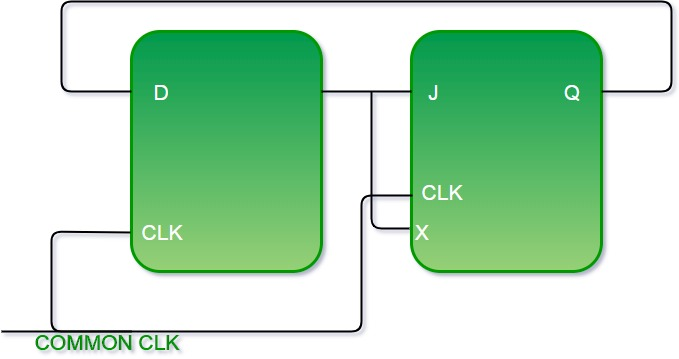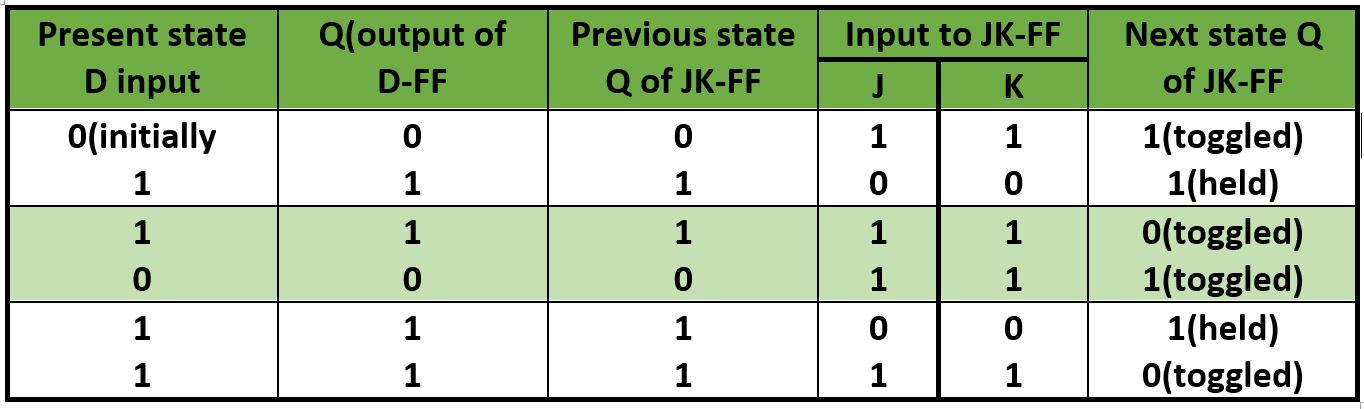Skip to content
Related Articles
GATE | GATE-CS-2015 (Set 1) | Question 47
• Last Updated : 19 Nov, 2018

A positive edge-triggered D flip-flop is connected to a positive edge-triggered JK flipflop as follows. The Q output of the D flip-flop is connected to both the J and K inputs of the JK flip-flop, while the Q output of the JK flip-flop is connected to the input of the D flip-flop. Initially, the output of the D flip-flop is set to logic one and the output of the JK flip-flop is cleared. Which one of the following is the bit sequence (including the initial state) generated at the Q output of the JK flip-flop when the flip-flops are connected to a free-running common clock? Assume that J = K = 1 is the toggle mode and J = K = 0 is the state-holding mode of the JK flip-flop. Both the flip-flops have non-zero propagation delays.
(A) 0110110…
(B) 0100100…
(C) 011101110…
(D) 011001100…

Answer: (A)

Explanation:Initially Q output of D – FF  = 1

Initially Q output of JK – FF  = 0

Now with the help of present state and next state table we can see what is happening in circuit.

• Toggle: J = K = 1
• Hold : J = K = 0We see from table Q output of D-FF is going to next state input of JK-FF and the bits sequence produced is like 110110…..

Including initial condition (0) we get output as 0110110110….

Hence answer is (A) part.

Another Explanation:

Here, it is given that JK flip flop will toggle when J = K = 1 and it will retain the output if J = K = 0.
Also, the output of the D flip flop would remain the same as the input.

So, we have

Initial output : D = 1

JK = 0

```After clock 1 : D = 0 (D gets 0 as input
from initial output of JK, so output = 0)```

JK = 1 (J = K = 1 from initial output of D, so output would be toggled from 0 to 1)

```After clock 2 : D = 1 (D gets 1 as input
from previous output of JK, so output = 1)```

JK = 1 (J = K = 0 from previous output of D, so output would be retained to 1)

```After clock 3 : D = 1 (D gets 1 as input
from previous output of JK, so output = 1)```

JK = 0 (J = K = 1 from previous output of D, so output would be toggled from 1 to 0)

```After clock 4 : D = 0 (D gets 0 as input
from previous output of JK, so output = 0)```

JK = 1 (J = K = 1 from previous output of D, so output would be toggled from 0 to 1)

```After clock 5 : D = 1 (D gets 1 as input
from previous output of JK, so output = 1)```

JK = 1 (J = K = 0 from previous output of D, so output would be retained to 1)

Thus, the bit sequence generated at the Q output of the JK flip flop will be 0110110…

Attention reader! Don’t stop learning now. Learn all GATE CS concepts with Free Live Classes on our youtube channel.

My Personal Notes arrow_drop_up